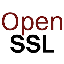OpenSSL "ans1parse -strparse" - Extract ASN.1 Sub Structure

Q

How to how extra an ASN.1 child structure using the OpenSSL "ans1parse" command?

✍: FYIcenter.com

AYou can use the OpenSSL "ans1parse -strparse offset" command to extract an ASN.1 child structure from a nested ASN.1 structure. as shown in the test below:

```C:\Users\fyicenter>\local\openssl\openssl.exe

OpenSSL> asn1parse -inform DER -in asn1_question.der -i
0:d=0  hl=2 l= 105 cons: SEQUENCE
2:d=1  hl=2 l=   1 prim:  INTEGER           :05
5:d=1  hl=2 l=  53 prim:  IA5STRING         :In asymmetric encryption,
which key can be given out?
60:d=1  hl=2 l=  45 cons:  SEQUENCE
62:d=2  hl=2 l=  14 prim:   IA5STRING         :A) Private key
78:d=2  hl=2 l=  13 prim:   IA5STRING         :B) Public key
93:d=2  hl=2 l=  12 prim:   IA5STRING         :C) Both keys

OpenSSL> asn1parse -inform DER -in asn1_question.der -i -strparse 60
0:d=0  hl=2 l=  45 cons: SEQUENCE
2:d=1  hl=2 l=  14 prim:  IA5STRING         :A) Private key
18:d=1  hl=2 l=  13 prim:  IA5STRING         :B) Public key
33:d=1  hl=2 l=  12 prim:  IA5STRING         :C) Both keys

OpenSSL> asn1parse -inform DER -in asn1_question.der -i -strparse 60
-noout -out asn1_question_option.der

OpenSSL> asn1parse -inform DER -in asn1_question_option.der
0:d=0  hl=2 l=  45 cons: SEQUENCE
2:d=1  hl=2 l=  14 prim: IA5STRING         :A) Private key
18:d=1  hl=2 l=  13 prim: IA5STRING         :B) Public key
33:d=1  hl=2 l=  12 prim: IA5STRING         :C) Both keys

OpenSSL> asn1parse -inform DER -in asn1_question_option.der -strparse 33
Error in encoding
12608:error:0D07209B:asn1 encoding routines:ASN1_get_object:too long:
.\crypto\asn1\asn1_lib.c:147:
```